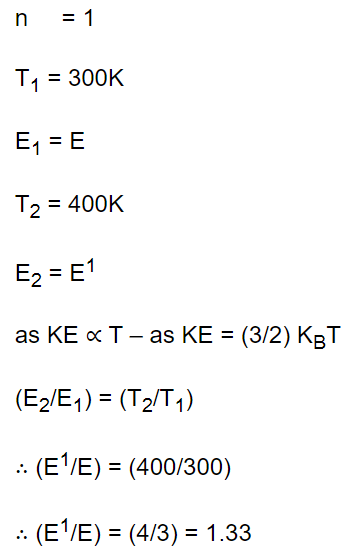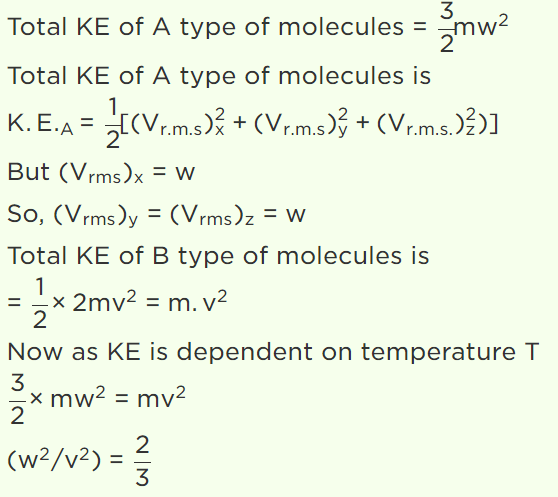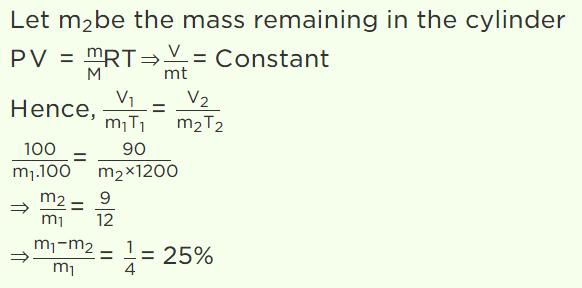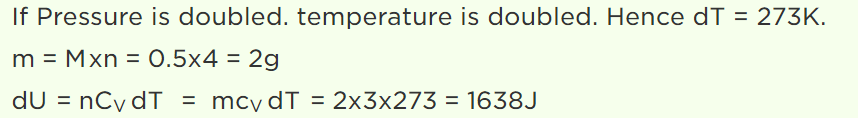## Kinetic Theory Questions and Answers Part-27

1. In kinetic theory of gases, which of the following statements regarding elastic collisions of the molecules is wrong
a) Kinetic energy is lost in collisions
b) Kinetic energy remains constant in collision
c) Momentum is conserved in collision
d) Pressure of the gas remains constant in collisions

Explanation: Kinetic energy is lost in collisions

2. If r.m.s. velocity of a gas is $V_{rms}=1840m\diagup s$    and its density $\rho=8.99\times 10^{-2}kg\diagup m^{3}$     the pressure of the gas will be
a) $1.01N\diagup m^{2}$
b) $1.01\times 10^{3}N\diagup m^{2}$
c) $1.01\times 10^{5}N\diagup m^{2}$
d) $1.01\times 10^{7}N\diagup m^{2}$

Explanation: $1.01\times 10^{5}N\diagup m^{2}$

3. An ideal gas is filled in a vessel, then
a) If it is placed inside a moving train, its temperature increases
b) Its centre of mass moves randomly
c) Its temperature remains constant in a moving car
d) None of these

Explanation: An ideal gas is filled in a vessel, then its temperature remains constant in a moving car

4. The kinetic energy of one mole gas at 300K temperature, is E. At 400K temperature kinetic energy is $E'$ . The value of $E'\diagup E$  is
a) 1.33
b) $\sqrt{\left(\frac{4}{3}\right)}$
c) $\frac{16}{9}$
d) 2

Explanation:5. N molecules each of mass m of gas A and 2N molecules each of mass 2m of gas B are contained in the same vessel at temperature T. The mean square of the velocity of molecules of gas B is $V^{2}$ and the mean square of x component of the velocity of molecules of gas A is $W^{2}$ . The ratio $\frac{W^{2}}{V^{2}}$
a) 1
b) 2
c) $\frac{1}{3}$
d) $\frac{2}{3}$

Explanation:6. A gas is filled in a cylinder, its temperature is increased by 20% on Kelvin scale and volume is reduced by 10%. How much percentage of the gas will leak out
a) 30%
b) 40%
c) 15%
d) 25%

Explanation:7. The air density at Mount Everest is less than that at the sea level. It is found by mountaineers that for one trip lasting a few hours, the extra oxygen needed by them corresponds to 30,000 cc at sea level (pressure 1 atmosphere, temperature 27°C). Assuming that the temperature around Mount Everest is –73°C and that the oxygen cylinder has capacity of 5.2 litre, the pressure at which $O_{2}$ be filled (at site) in cylinder is
a) 3.86 atm
b) 5.00 atm
c) 5.77 atm
d) 1 atm

Explanation:8. $\frac{1}{2} mole$   of helium gas is contained in a container at S.T.P. The heat energy needed to double the pressure of the gas, keeping the volume constant (specific heat of the gas $=3 J gm^{-1}K^{-1}$   )
a) 3276 J
b) 1638 J
c) 819 J
d) 409.5 J

Explanation:9. The equation of state of a gas is given by $\left(P+\frac{aT^{2}}{V}\right)V^{C}=\left(RT+b\right)$     , where a, b, c and R are constants. The isotherms can be represented by $P=AV^{m}-BV^{n}$   , where A and B depend only on temperature and
a) m=-c and n=-1
b) m=c and n=1
c) m=-c and n=1
d) m=c and n=-1